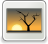# Non-dimensional system for analysis equilibrium point mathematical model of tumor growth

Mustafa, Mamat and Subiyanto, Subiyanto and Mohammad Fadhli, Ahmad (2014) Non-dimensional system for analysis equilibrium point mathematical model of tumor growth. Applied Mathematical Sciences, 8 (2). pp. 91-98. ISSN 1312885X [P]Image FH02-FIK-14-00727.jpg Restricted to Registered users only Download (187kB)

## Abstract

In this paper we presented analysis equilibrium point of mathematical model tumor growth use non-dimensional technique. This technique is useful to simply equation that complicated as a mathematical model that present in this paper. This mathematical model describes the effect of tumor infiltrating lymphocytes (TIL) and interleukin-2 (IL-2) on the dynamics of tumor cells. The steps of this technique are presented. With this technique we can be to determine more accurately the equilibria of our system which form nonlinear dynamics of system ordinary differential equation that coupled.

Item Type: Article equilibrium point, interleukin, non-dimensional, tumor infiltrating lymphocytes, tumor growth Q Science > QA Mathematics Faculty of Informatics & Computing Syahmi Manaf 13 Sep 2022 05:18 13 Sep 2022 05:18 http://eprints.unisza.edu.my/id/eprint/4933View Item